# Chapter 4.2. More complex conditions – Exam tasks

In the previous chapter, we got familiar with the nested conditional constructions in the Java language. Through them, the logic in a program can be represented by if conditional statements that are nested in each other. We also looked at the conditional switch-case construction, which allows you to choose from a list of options. We should practice and consolidate what we have learned so far by looking at a few more complex exam tasks. Before moving on to the tasks, we will recall the conditional constructions:

## Nested conditions

if (condition1) {
if (condition2) {
// body of if construction
} else {
// body of else construction
}
}Remember that it is not a good practice to write deeply nested conditional statements (with more than three levels of nesting). Avoid nesting of more than three conditional statements inside one another. This complicates the code and makes its reading and understanding difficult.

## Switch-case conditions

When the program operation depends on the value of a variable, instead of doing consecutive checks with multiple if-else blocks, we can use the switch-case conditional statement.

switch (selector) {
case value1:
//code to be executed
break;
case value2:
//code to be executed
break;
default:
//code to be executed
break;
}


The structure consists of:

• Selector - an expression that is calculated to some specific value. The type of the selector can be an integer, string or enum.
• Multiple case labels followed by code to be executed, ending with break.

Now that we have recalled how conditional statements are used and nested into each other to implement more complex conditions and program logic, let's solve a few exam tasks.

## Task: On Time for the exam

A student must go to the exam at a certain time (for example at 9:30am). He arrives at the exam hall at a given hour of arrival (for example 9:40). It is considered that the student has arrived on time, if he arrives at the time when the exam starts or up to half an hour earlier. If the student arrives more than 30 minutes earlier, the student has come too early. If he arrives after the time when the exam starts, he is late.

Write a program that has as input data for the exam starting time and the student arrival time, and prints if the student has arrive on time, or if he has arrive early or if he is late, as well as how many hours or minutes the student was early or late.

### Input data

From the console, we read as input data four integers (one per line):

• The first line contains exam starting time(hours) – an integer from 0 to 23.
• The second line contains exam starting time(minutes) – an integer from 0 to 59.
• The third line contains hour of arrival – an integer from 0 to 23.
• The fourth line contains minutes of arrival – an integer from 0 to 59.

### Output data

Print on the first line:

• "Late", if the student arrives later than the exam starting time.
• "On time", if the student arrives on time exactly at the exam starting time or up to 30 minutes earlier.
• "Early", if the student arrives more than 30 minutes before the exam starting time.

If the student arrives with more than one minute difference compared to the exam starting time, print on the next line:

• "mm minutes before the start" for arriving less than an hour earlier.
• "hh:mm hours before the start" for arriving 1 hour or earlier. Always print the minutes using two digits, for example, "1:05".
• "mm minutes after the start" for arriving less than an hour late.
• "hh:mm hours after the start" for arriving late with 1 hour or more. Always print the minutes using two digits, for example, "1:03".

### Sample input and output

Input Output Input Output
9
30
9
50
Late
20 minutes after the start
16
00
15
00
Early
1:00 hours before the start
9
00
8
30
On time
30 minutes before the start
9
00
10
30
Late
1:30 hours after the start
14
00
13
55
On time
5 minutes before the start
11
30
8
12
Early
3:18 hours before the start
Input Output
10
00
10
00
On time
11
30
10
55
Early
35 minutes before the start
11
30
12
29
Late
59 minutes after the start

### Hints and guidelinesIt is recommended that you read the condition of a task several times taking notes and sketching examples while thinking about them, before you start writing code.

#### Input data processing

According to the assignment, we expect four lines in a row with different integers. Looking at the provided parameters, we can use the type int, as it is suitable for the expected values. We simultaneously read an input and parse the string value to the selected data type for integer.Looking at the expected output, we can create variables that contain the different output data types to avoid using the so-called "magic strings" in the code.#### Calculations

After reading the input data, we can now start writing the logic for calculating the result. Let's first calculate the starting time of the exam in minutes for easier and more accurate comparison.Let's also calculate the arrival time of the student.It remains for us to calculate the difference between the two times, to determine when and what time compared to the exam time the student has arrived.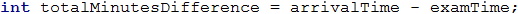Our next step is to do the required checks and calculations, and finally, we will print the output. Let's separate the code into two parts:

• First, let's show when the student arrived – is he early, late or on time. Тo do that, we will use an if-else statement.
• After that, we will show the time difference if the student arrives in a different time from the starting time of the exam.

To spare one additional check (else), we can, by default, assume that the student was late.

According to the condition, we will check whether the difference in times is more than 30 minutes. If this is true, we assume that the student is early. If we do not match the first condition, we need to check if the difference is less than or equal to zero (<= 0), by which we are checking the condition whether the student arrived within the range of 0 to 30 minutes before the exam.

In all other cases, we assume that the student is late, which we did by default. Therefore, no additional check is needed.Finally, we need to find out and print what is the time difference between exam start time and student arrival time. Also, whether this difference shows the time of arrival before or after the exam start.

We also check whether the time difference is more than one hour, to print hours and minutes in the required format, or if it is less than one hour, to print only minutes in the required format and description.

We also need to do one more check – whether the time of the student arrival is before or after the exam start time.#### Printing the result

Finally, it remains to print the result on the console. According to the requirements, if the student arrived right in time (not even a minute difference), we do not need to print a second result. This is why we do the following check: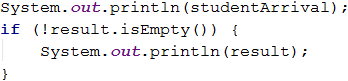Printing the result on the console can be done at a much earlier stage – during the calculations. This, however, is not a very good practice. Why?

Let's look at the idea that our code is not 10 lines, but 100 or 1000! One day, printing the result will not be done on the console, but will be written in a file or displayed as a web aplication. Then, on how many places in the code we need to do changes? Are you sure you won't miss some places?Always consider the code that contains logical calculations as a separate part different from the part that processes the input and output data. It must be able to work regardless of how the data is passed to it and where the result will be displayed.

### Testing in the Judge system

Most people plan their vacations. A young programmer has a fixed budget and spare time in a particular season.

Write a program that accepts as an input the budget and the season, and as an output to print where the programmer will rest and the amount of money he will spend.

The budget determines the destination and the season what amount of the budget will be spent. If the season is summer, he will go camping, and if it is winter - in a hotel. If it is in Europe, regardless of the season, the programmer will stay in a hotel. Each camping or hotel, according to the destination, has its price, which corresponds to a particular percentage of the budget:

• If 100 BGN or less – somewhere in Bulgaria.
• Summer30% of the budget.
• Winter70% of the budget.
• If 1000 BGN or less – somewhere on the Balkans.
• Summer40% of the budget.
• Winter80% of the budget.
• If more than 1000 BGN. – somewhere in Europe.
• Upon traveling in Europe, regardless of the season, the programmer will spend 90% of the budget.

### Input data

The input data will be read from the console and will consist of two lines:

• On the first line we receive the budget - a floating-point number int the range of [10.00 … 5000.00].
• On the second line – one of the two possible seasons: "summer" or "winter".

### Output data

On the console must be printed two lines.

• On the first line – "Somewhere in {destination}", where {destination} is "Bulgaria", "Balkans" or "Europe".
• On the second line – "{Vacation type} – {Spent money}":
• The vacation can be "Camp" or "Hotel".
• The amount of money has to be rounded up to the second digit after the decimal point.

### Sample Input and Output

Input Output
50
summer
Somewhere in Bulgaria
Camp - 15.00
75
winter
Somewhere in Bulgaria
Hotel - 52.50
312
summer
Somewhere in Balkans
Camp - 124.80
678.53
winter
Somewhere in Balkans
Hotel - 542.82
1500
summer
Somewhere in Europe
Hotel - 1350.00

### Hints and guidelines

Like the other tasks, we can separate the solution of our task into the following parts: reading the input data, doing calculations, printing the result.

#### Input data processing

While carefully reading the requirements, we understand that we expect two lines of input data. The first parameter is a real number, for which we need to pick an appropriate variable type. For a higher level of calculation accuracy, we can pick BigDecimal as a type for the budget and string for the season.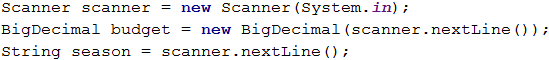Always consider, what is the **type** in the input data, as well as to what data type they need to be converted to, so created program constructs to work correctly!

Example: When you need to do calculations related to money, use BigDecimal for a higher level of accuracy.

#### Calculations

Let's create and initialize the variables needed for applying the logic and calculations.Similar to the example in the previous task, we can initialize variables with some of the output results, in order to spare additional initialization.

When examining once again the requirements, we notice that the decision of where the vacation will take place depends on the amount of the budget we receive as input data, i.e., in our logic, we have two cases:

• If the budget is less than or equals a particular value.
• If it is less than or equals other value or is more than the specified border value.

Based on the way we arrange the logical scheme (the order in which we will check the border values), we will have more or fewer conditions in the solution. Think why!

After that, we need to check the value of the given season. Based on the result, we will determine what percentage of the budget will use, and where the programmer will stay – in a hotel or a camp.

Let's choose and create the needed format for the output data, which we will use further in the code:

DecimalFormat formatter = new DecimalFormat("0.00");


Here is a sample code that we can use to implement the described logic above:After, we continue with:and finish the conditions with: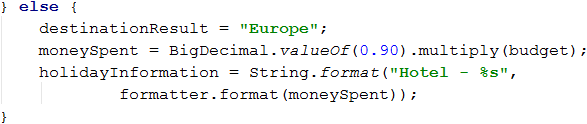We always can initialize the variable with a given value and then make only one check. This saves us a logic step.

For example, the following code:can be shortened to this: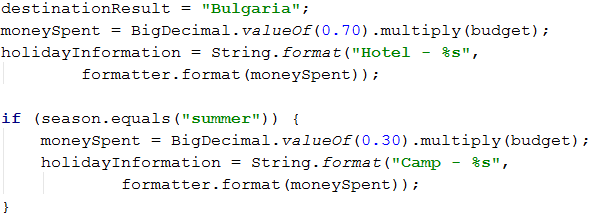#### Printing the result

It remains to print the obtained result on the console: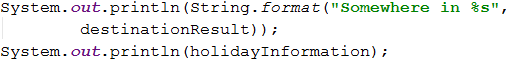### Testing in the Judge system

Write a program that reads as input data two integers (N1 и N2) and an operator that performs a particular mathematical operation with them. Possible operations are: addition (+), subtraction (-), multiplication (*), division (/) and modular division (%). Upon adding, subtracting, and multiplying, print on the console the result and display whether it is even or odd. In simple division – only the result, and in the modular division – the remainder. You need to take into account that the divisor can be equal to zero (= 0), and dividing by zero is not possible. In this case, print a special message.

### Input data

The input data will be read from the console and will consist of three lines:

• On the first line N1integer within the range [0 … 40 000].
• On the second line N2integer within the range [0 … 40 000].
• On the third line Operatorone character among: "+", "-", "*", "/", "%".

### Output data

Print one line on the console:

• If the operation is addition, subtraction or multiplying:
• "{N1} {operator} {N2} = {output} – {even/odd}".
• If the operation is division:
• "{N1} / {N2} = {output}" – the result is formatted to the second digit after the decimal point.
• If the operation is modular division:
• "{N1} % {N2} = {remainder}".
• In case of dividing by 0 (zero):
• "Cannot divide {N1} by zero".

### Sample input and output

Input Output Input Output
123
12
/
123 / 12 = 10.25 112
0
/
Cannot divide 112 by zero
10
3
%
10 % 3 = 1 10
0
%
Cannot divide 10 by zero
Input Output
10
12
+
10 + 12 = 22 - even
10
1
-
10 - 1 = 9 - odd
7
3
*
7 * 3 = 21 - odd

### Hints and guidelines

The task is not complicated, but there are many lines of code to write.

#### Input data processing

After reading the condition, we understand that we expect three lines of input data. On the first two lines we expect two integers (within the specified range), and on the third line - an arithmetical symbol.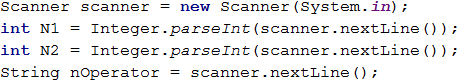#### Calculations

Let's create and initialize the variables needed for the logic and calculations. In one variable, we will store the result from the calculations, and in the other one, we will use it for the final output of the program.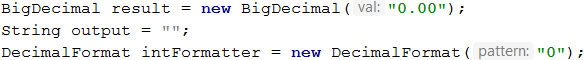Reading the condition carefully, we understand that there are cases where we don't need to do any calculations but simply print the result.

Therefore, we can first check if the second number is 0 (zero), whether the operation is division or modular division, and then initialize the output.Let's put the result as a value upon initializing the output parameter. This way we, can make only one check - whether it is necessary to recalculate and replace this result.

Based on the approach that we choose, our next check will be either a simple else or a single if. In the body of this check, using additional checks, according to the submitted operator, we can divide the logic according to the structure of the expected output.

From the condition we can see that for addition (+), subtraction (-) or multiplication (*) the expected output has the same structure: "{N1} {operator} {N2} = {output} – {even/odd}", whereas for division (/) and modular division (%) the output has a different structure.We finish the solution by applying conditions for addition, subtraction and multiplication: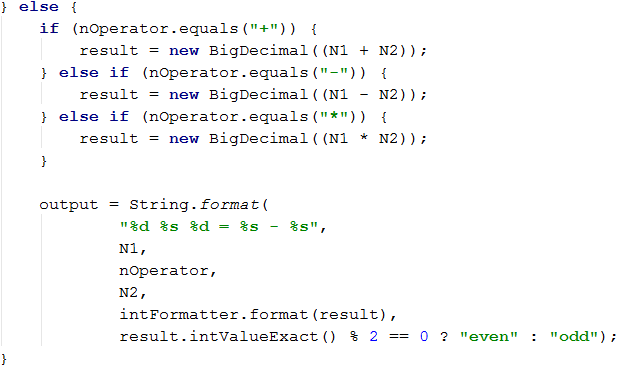For short and clear check, such as the above example for even and odd numbers, you can use a ternary operator. Let's look at the possible check with and without ternary operator.

Without using a ternary operator the code is longer but easy to read:Upon using a ternary operator the code is much shorter but may require additional efforts to read and understand the logic: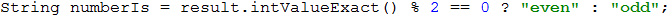#### Printing the output

Finally, we need to show the calculated result to the console: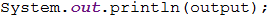### Testing in the Judge system

A group of football fans decided to buy tickets for Euro 2016. The ticket price is determined according to two categories:

• VIP499.99 BGN
• Normal249.99 BGN

The fans have a certain budget, and the number of people in the group determines what percentage of the budget should be set aside for transport:

• From 1 to 4 – 75% of the budget
• From 5 to 9 – 60% of the budget
• From 10 to 24 – 50% of the budget
• From 25 to 49 – 40% of the budget
• 50 or more – 25% of the budget

Write a program that calculates, whether with the rest money from the budget they can buy tickets for the selected category, as well as how much money they will have left or will need.

### Input data

The input is read from the console and contains exactly 3 lines:

• On the first line is the budget – a floating-point number in the range of [1 000.00 … 1 000 000.00].
• On the second line is the category – "VIP" or "Normal".
• On the third line is the number of people in the group – an integer in the range of [1 … 200].

### Output data

Print one line on the console:

• If the budget is enough:
• "Yes! You have {N} leva left." – where N is the remaining amount of the group.
• If the budget is not enough:
• "Not enough money! You need {М} leva." – where М is the amount that is insufficient.

The amounts must be formatted up to the second digit after the decimal point.

### Sample input and output

Input Output Explanations
1000
Normal
1
Yes! You have 0.01 leva left. 1 person: 75% of the budget are spent on transport.
Remaining amount: 1000 – 750 = 250.
Category Normal: The ticket price is 249.99 * 1 = 249.99
249.99 < 250: they remain to him 250 – 249.99 = 0.01
Input Output Explanations
30000
VIP
49
Not enough money! You need 6499.51 leva. 49 people: 40% of the budget are spent on transport.
Remaining amount: 30000 – 12000 = 18000.
Category VIP: The ticket price is 499.99 49.
24499.510000000002 < 18000.
Money needed 24499.51 - 18000 = *6499.51

### Hints and guidelines

We will read the input data and do the calculations described in the condition to check if the money will be sufficient.

#### Input data processing

Let's read the condition carefully and look at what we expect to get as an input data, what we expect to return, as a result,, and what are the main steps when dividing the logic.

To begin with, let's process and save the input data in appropriate variables:#### Calculations

Let's create and initialize the needed variables for the calculations:Let's review the condition again. We need to do two different calculations.

The first calculations are about what part of the budget we will have to set aside for transport. For the logic of these calculations, we will notice that only the number of people in the group matters. Therefore, we will make a logical breakdown by the number of fans.

We will use a conditional construction - a series of if-else blocks.The second calculations are about what amount we will need to buy tickets for the group. According to the condition, it depends only on the type of tickets, we have to buy.

Let's use switch-case conditional construction.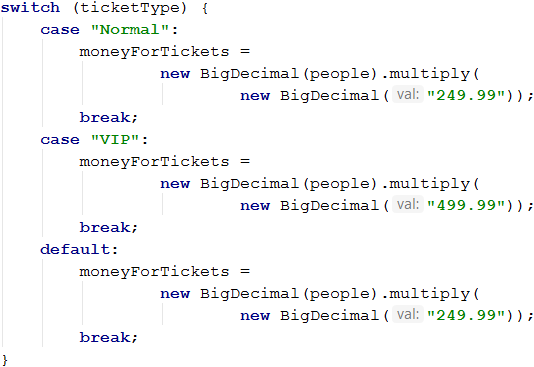Once we have calculated the transport and the tickets costs, what remains is to calculate the final result and see whether it will succeed the group of fans to attend the Euro 2016 or not with these parameters given.

For printing the result, in order to skip one else condition, we will assume that the group can, by default, attend Euro 2016.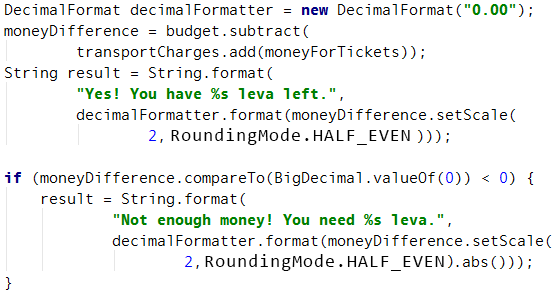#### Printing the result

Finally, we need to print the calculated result on the console.

### Testing in the Judge system

A hotel offers two types of rooms: studio and apartment.

Write a program that calculates the price of the entire stay for a studio and apartment. The prices depend on the month of the accommodation:

May and October June and September July and August
Studio – 50 BGN/per night Studio – 75.20 BGN/per night Studio – 76 BGN/per night
Apartment – 65 BGN/per night Apartment – 68.70 BGN/per night Apartment – 77 BGN/per night

The following discounts are offered:

• For studio, in case of more than 7 nights in May and October: 5% discount.
• For studio, in case of more than 14 nights in May and October: 30% discount.
• For studio, in case of more than 14 nights in June and September: 20% discount.
• For apartment, in case of more than 14 nights, no matter the month: 10% discount.

### Input data

The input data is read from the console and contains exactly two lines:

• On the first line is the month – May, June, July, August, September, or October.
• On the second line is the number of nights – integer in the range of [0 … 200].

### Output data

Print two lines on the console:

• On the first line: "Apartment: { price for the whole stay } BGN"
• On the second line: "Studio: { price for the whole stay } BGN"

The price for the entire stay must be formatted up to two digits after the decimal point.

### Sample input and output

May
15
Apartment: 877.50 BGN
Studio: 525.00 BGN
In may, for more than 14 nights, we decrease the price of the studio with 30% (50 – 15 = 35), and for the apartment – with 10% (65 – 6.5 =58.5).
The entire stay in the apartment – 877.50 BGN
The entire stay in the studio – 525.00 BGN
Input Output
June
14
Apartment: 961.80 BGN
Studio: 1052.80 BGN
August
20
Apartment: 1386.00 BGN
Studio: 1520.00 BGN

### Hints and guidelines

First, we will read the input data and then perform the calculations according to the provided price list and the discount rules. Finally print the result.

#### Input data processing

According to the condition, we expect to read two lines of input data - on the first line he month in which the stay is planned, on the second - the number of nights.

Let's process and store the input data in appropriate variables:#### Calculations

Let's create and initialize the variables needed for the calculations:Looking again at the condition, we notice that our basic logic depends on what month we receive as input data and the number of nights.

In general, there are different approaches and ways to make the checks in question, but let's focus on the switch-case construction, and in the different case blocks, we will use conditional statements such as if and if-else.

Let's start with the first group of months: May and October. For these two months the price for the stay is the same for both types of accommodation - studio and apartment. Therefore, it is necessary to make an internal check against the number of nights to recalculate the corresponding price (if needed).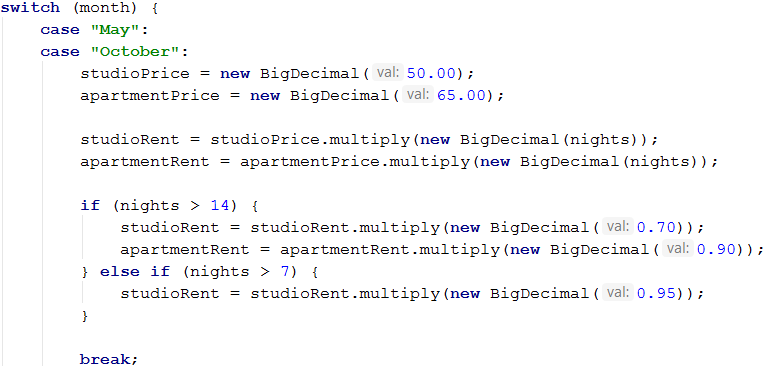For the following months, the logic and the calculations are identical.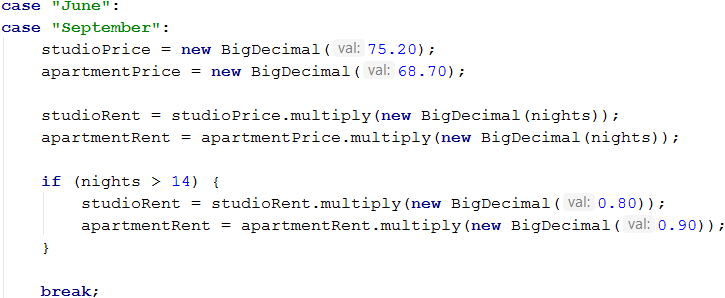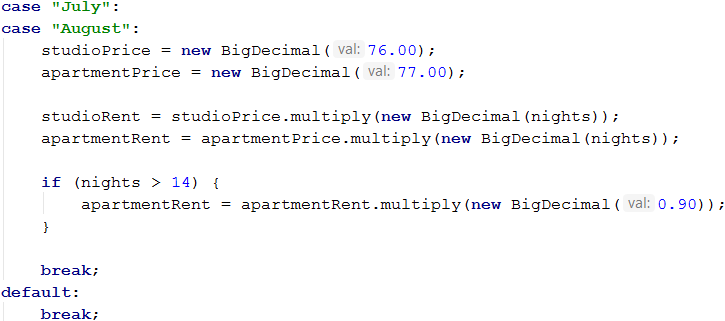After calculating the relevant prices and the total amount for the stay - let's prepare the formatted result. Before that, we should store it in our output variables - studioInfo and apartmentInfo.To calculate the output parameters, we use the method java.math.BigDecimal.setScale(int newScale, RoundingMode roundingMode). This method rounds a decimal number to a specified number of digits after the decimal point, and we have control of any rounding type - RoundingMode. For this purpose, we give the method an integer (int) with which we indicate till which sign we want to round the number and value of a given enumeration RoundingMode (UP, DOWN, CEILING, FLOOR, HALF_UP, HALF_DOWN, HALF_EVEN). In our case, we will round the decimal number up to two digits after the decimal point with rounding type HALF_UP.

#### Printing the result

Finally, what remains is to print the calculated results on the console.